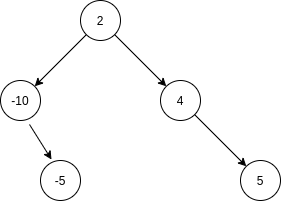# Minimal Tree

Posted: 26 Feb, 2021
Difficulty: Easy

## PROBLEM STATEMENT

#### Note :

``````1. There can be more than one way to convert an array to a height-balanced binary tree. You can find any one of them.
``````

#### Example :

``````Consider an array ‘Arr’ = [-10, -5, 2, 4, 5],  one way to convert it to a height-balanced binary tree is -:
````````````Here, You can see that the height of the left and right subtree of the node having the data ‘2’ is  2 and 2 respectively, i.e both are the same,  and the height of the left and the right tree of the node having the data ‘-10’, is 0, 1 respectively, i.e differ by only 1,  and the height of left and right subtree of the node having the data ‘4’, is also 0 and ‘1’ respectively, i.e differ by only ‘1’. Thus this binary search tree is height-balanced.  Also, note that this is not the only way to convert this array to a height-balanced binary search tree.
``````Approach 1

The root of the height-balanced binary search tree will be the middle element of the given array, and its left subtree will be the height-balanced binary search formed by the elements at the left of the middle, and its right subtree will be the height-balanced binary search formed by the elements at the right of the middle elements. So, we can form tree recursively as follows:

Algorithm

• Initialize two integer variables ‘left’ := 0 and ‘right’ := N-1.
• Create a recursive function minimalTreeHelper(left, right) and in each recursive call do the following:
• If left > right, then return NULL.
• Initialize an integer variable mid:= (left + right)/2.
• Create a node of a binary tree having the data Arr[mid] and make it the root of this tree.
• The left subtree of the root will be returned by minimalTreeHelper(left, mid-1) and the right subtree of the node will be returned by minimalTreeHelper(mid+1, right).
• Return root.
• The required height-balanced binary search tree will be returned by minimalTreeHelper(0, N-1).